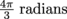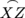# SAT Math: Calculating Arc Length Using Proportions

A circle has a radius of 6 and a central angle ∠XYZ that measures. What is the length of the major arcformed by the central angle?

 Additional Topics in Math Congruence and similarity Product Type SAT Math SAT SAT Math SAT Math Additional Topics in MathGeometry and Measurement Test Prep SAT Math

### Transcript

00:19

here It's gonna look like this I'm here to here's

00:22

to forty And then we got to X and line

00:24

Zeke And yes how rude it was of this problem

00:27

to not come with a drawing Yeah you have to

00:29

make one sometimes but that's because we're in America No

00:32

00:35

of four pi over three and converted to degrees We

00:39

had for pirating ins over three times the hundred degrees

00:42

over pi radiance That's how we got to two Forty

00:44

They're spinning counterclockwise Okay And this is how big the

00:48

central angle in the circle has to be This thing

00:51

which is a pretty big to forty big number Imagine

00:54

having to run around the circle to get from X

00:55

to Z by taking the longer route Well that's the

00:58

root of the major ark We need to measure and

01:00

to find the distance around a part of the circle

01:03

First find the distance around the full circle which is

01:05

the What's it called Oh yes Circumference Well the problem

01:08

says the radius is six and the circumference is always

01:11

two Pi r Right That's just by definition So sir

01:14

Come sir Go to buy our equals two high times

01:17

six which is twelve Pie Got it Simple That's the

01:20

circumference Well given the circumference here we can measure the

01:23

art by setting up a proportion We need to measure

01:25

the arc length created by two hundred forty degree arc

01:28

out of the full circle Three Sixty measure So what

01:31

is that Well we got to forty over three Sixty

01:33

There equals that arc x z over twelve pie because

01:37

that's a circumference right That's the three sixty Think so

01:40

reduces down to two thirds equals xy over twelve pie

01:43

Or send another way If we divide the numbers out

01:45

here we get well the ark XY is just a

01:48

pie So the length of the major arc X Z

01:51

formed by the central angle is a pirate's answer See

01:54

and remember this is a distance of the long way

01:57

From ecstasy not the short way which is for pie

02:00

They throw that curve ball like you There'd be and

02:02

twelve five's The total circumference on a portion of it

02:05

into pie is the full angle of a circle and

02:07